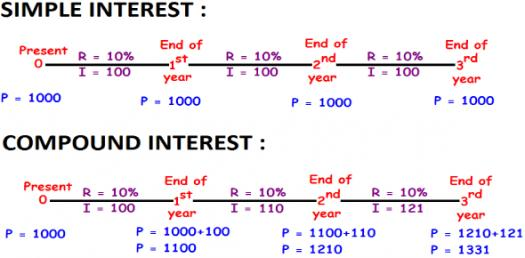# Chapter 7: Simple Interest (Assume 360 Days In One Year)

Approved & Edited by ProProfs Editorial Team
The editorial team at ProProfs Quizzes consists of a select group of subject experts, trivia writers, and quiz masters who have authored over 10,000 quizzes taken by more than 100 million users. This team includes our in-house seasoned quiz moderators and subject matter experts. Our editorial experts, spread across the world, are rigorously trained using our comprehensive guidelines to ensure that you receive the highest quality quizzes.
| By Rmcgowan
R
Rmcgowan
Community Contributor
Quizzes Created: 4 | Total Attempts: 3,350
Questions: 7 | Attempts: 306SettingsThis is a quiz to test students' knowledge of simple interest.

• 1.

### Suppose \$4500 is invested for 60 days at an annual rate of 6%. How much interest will be earned? Include the \$ in front of your answer.

Explanation
The formula for calculating simple interest is: Interest = Principal x Rate x Time. In this case, the principal is \$4500, the rate is 6% (or 0.06 as a decimal), and the time is 60 days (or 60/365 as a fraction of a year). Plugging in these values into the formula, we get: Interest = \$4500 x 0.06 x (60/365) = \$44.38. Therefore, the correct answer is \$44.38.

Rate this question:

• 2.

### Mr. McGowan borrowed \$840 for 60 days at 9% interest. However, he was able to repay the loan in 30 days. How much interest was he able to save by doing this? Include the \$ in front of your answer.

Explanation
Mr. McGowan borrowed \$840 for 60 days at 9% interest. However, he was able to repay the loan in 30 days. The interest he would have paid for the full 60 days can be calculated using the formula: interest = principal * rate * time. Plugging in the values, we get interest = \$840 * 9% * 60/365 = \$11.01. Since he repaid the loan in 30 days instead, the interest he actually paid is \$840 * 9% * 30/365 = \$4.71. Therefore, the amount of interest he saved by repaying the loan early is \$11.01 - \$4.71 = \$6.30.

Rate this question:

• 3.

### An automobile dealer borrowed \$180 000 from the bank at 6.5% annual interest. How much will he be charged for 270 days? Include the \$ in front of your answer.

Explanation
The automobile dealer borrowed \$180,000 from the bank at an annual interest rate of 6.5%. To calculate the amount charged for 270 days, we need to find the interest accrued during this period. The formula for calculating simple interest is: Interest = Principal * Rate * Time. Plugging in the values, we get: Interest = \$180,000 * 6.5% * (270/365) = \$8775. Therefore, the dealer will be charged \$8775 for 270 days.

Rate this question:

• 4.

### Interest on a 120-day loan of \$36000 is charged at an annual rate of 10%. How much interest is charged? Include the \$ in front of your answer.

Explanation
The correct answer is \$1200. The interest on a 120-day loan of \$36000 at an annual rate of 10% can be calculated using the formula: Interest = Principal x Rate x Time. In this case, the Principal is \$36000, the Rate is 10% (0.10 as a decimal), and the Time is 120 days divided by 365 (approximately 0.3288). Plugging these values into the formula, we get: Interest = \$36000 x 0.10 x 0.3288 = \$1183.56. However, since the question asks for the answer to be in dollars, we round up to the nearest dollar, resulting in \$1200.

Rate this question:

• 5.

### Suppose \$1800 is invested for 90 days at an annual rate of 6.5%. How much interest will be earned? Include the \$ in front of your answer.

Explanation
The correct answer is \$29.25. To calculate the interest earned, we can use the formula: Interest = Principal x Rate x Time. In this case, the principal is \$1800, the rate is 6.5% (or 0.065 as a decimal), and the time is 90 days (or 90/365 years). Plugging in these values, we get: Interest = \$1800 x 0.065 x (90/365) = \$29.25.

Rate this question:

• 6.

### What is 5 1/4 % as a fraction in lowest terms?

• A.

21/400

• B.

525/100

• C.

525/10000

• D.

5.25/100

• E.

21/4

A. 21/400
Explanation
To convert a mixed number to a fraction, we first convert the whole number part to a fraction by multiplying it by the denominator of the fraction and adding the numerator. In this case, 5 multiplied by 4 is 20, and adding 1 gives us 21. So, 5 1/4 can be written as 21/4. To find the percentage as a fraction, we divide the fraction by 100. Therefore, 5 1/4 % as a fraction in lowest terms is 21/400.

Rate this question:

• 7.

### What is 8 months in years?

• A.

.67 years

• B.

.8 years

• C.

8/11 years

• D.

.75 years

• E.

8/360 years

A. .67 years
Explanation
8 months is equivalent to 0.67 years. This can be calculated by dividing the number of months by 12, since there are 12 months in a year. In this case, 8 divided by 12 equals 0.67.

Rate this question:

Related TopicsBack to top